Tamilnadu State Board New Syllabus Samacheer Kalvi 12th Maths Guide Pdf Chapter 10 Ordinary Differential Equations Ex 10.7 Textbook Questions and Answers, Notes.

## Tamilnadu Samacheer Kalvi 12th Maths Solutions Chapter 10 Ordinary Differential Equations Ex 10.7

Question 1.
Solve the following Linear differential equations.
cos x $$\frac { dy }{ dx }$$ + y sin x = 1
Solution:
The given differential equation can be written as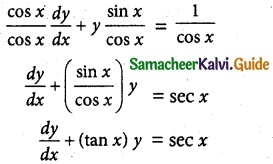This is the form $$\frac { dy }{ dx }$$ + py = Q
where P = tan x
Q = sec x
Thus, the given differential equation is linear.
I.F = e∫ pdx = e∫ tan x dx = elog (sec x) = sec x
So, the required solution is given by
[y × I.F] = ∫ [Q × IF] dx + c
y × sec x = ∫ sec x × sec x dx + c
y sec x = ∫ sec² x dx + c
y sec x = tan x + c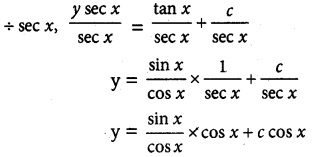= sin x + c cos x
y = sin x + c cos x is the required solution.Question 2.
(1 – x²)$$\frac { dy }{ dx }$$ – xy = 1
Solution:
The given differential equation can be written as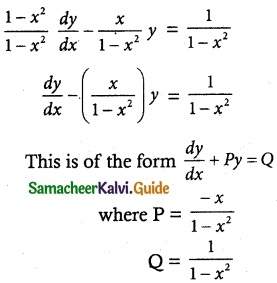Thus, the given differential equation is linear.Which is a required solution.Question 3.
$$\frac { dy }{ dx }$$ + $$\frac { y }{ x }$$ = sin x
Solution:
The given differential equation can be written as
$$\frac { dy }{ dx }$$ + ($$\frac { 1 }{ x }$$)y = sin x
This is of the form $$\frac { dy }{ dx }$$ + Py = Q
where P = $$\frac { 1 }{ x }$$
Q = sin x
Thus, the given differential equation is linear.
I.F= e∫ pdx = e∫ $$\frac { 1 }{ x }$$ dx = elog x = x
So, the required solution is given by
yx I.F = ∫ (Q × I.F) dx + c
yx = ∫ sin x × x dx + c
= x (-cos x) – (1) (-sin x) + c
yx = -x cos x + sin x + c
yx + x cos x = sin x + c
(y + cos x) x = sin x + c is a required solution.Question 4.
(x² + 1)$$\frac { dy }{ dx }$$ + 2xy = $$\sqrt { x^2+4 }$$
Solution:
The given differential equation may be written asThus, the given differential equation is linear.
I.F= e∫ pdx = e∫ $$\frac { 2x }{ x^+1 }$$ dx = elog (x² + 2) = x² + 1
So, the required solution is given byIs the required solution.

Question 5.
(2x – 10y³) dy + y dx = 0
Solution:
The given differential equation may be written as
y dx = -(2x – 10y³) dy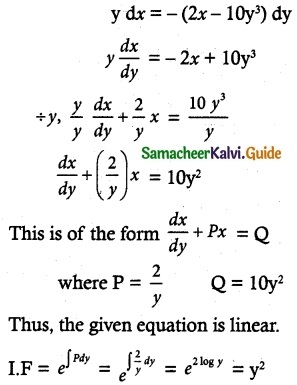So, the required solution is
x × I.F = ∫ (Q × I.F)dy + c
xy² = ∫ 10 y² × y² dy + c
= ∫ 10 y4 dy + c
= $$\frac { 10y^5 }{ 5 }$$ + c = 2y5 + c
xy² = 2y5 + c is a required solution.Question 6.
x sin x $$\frac { dy }{ dx }$$ + (x cos x + sin x) y = sin x
Solution:
The given differential equation can be written as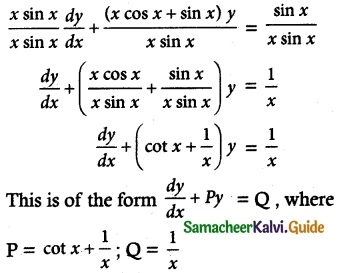Thus, the given differential equation is linear.
I.F = e∫ pdx = e∫ (cot x + 1/x)dx = elog sin x + log x
= elog (x sin x) = x sin x
So, the solution of the given differential equation is given by
y × I.F = ∫(Q × I.F) dx + c
y (x sin x) = ∫ $$\frac { 1 }{ x }$$ x sin x dx + c
= ∫ sin x dx + c
y (x sin x) = -cos x + c
xy sin x + cos x = c is the required solution.Question 7.
Solve (y – esin-1x) $$\frac { dx }{ dy }$$ + $$\sqrt { 1-x^2 }$$ = 0
Solution:Thus, the given differential equation is Linear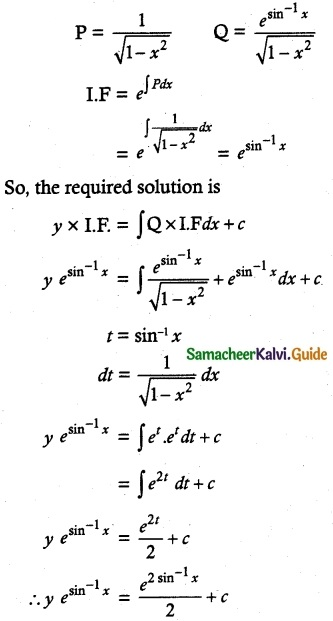Question 8.
$$\frac { dy }{ dx }$$ + $$\frac { y }{ (1-x)√x }$$ = 1 – √x
Solution:
The given linear differential equation is of the form
$$\frac { dy }{ dx }$$ + py = Q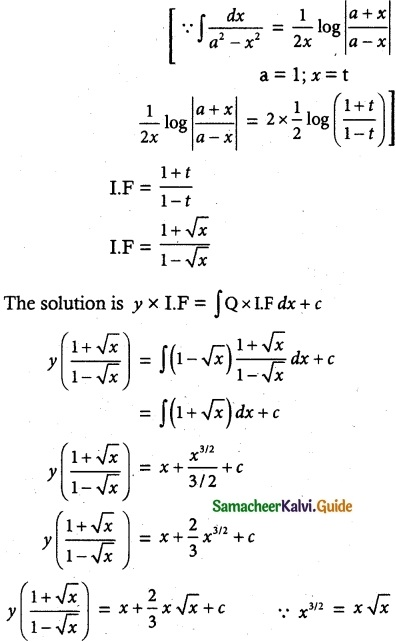Question 9.
(1 + x + xy²) $$\frac { dy }{ dx }$$ + (y + y³) = 0
Solution: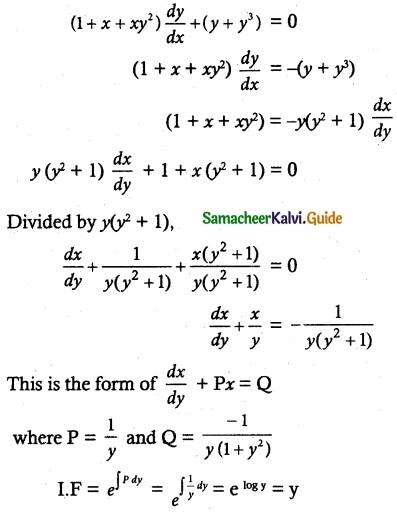So, the solution of the equation is given byxy + tan-1 y = c
Which is the required solution.

Question 10.
$$\frac { dy }{ dx }$$ + $$\frac { y }{ x log x }$$ = $$\frac { sin 2x }{ log x }$$
Solution:
The given differential equation may be written as
$$\frac { dy }{ dx }$$ + $$\frac { 1 }{ x log x }$$y = $$\frac { sin 2x }{ log x }$$
This is of the form $$\frac { dy }{ dx }$$ + Py = Q
Where P = $$\frac { 1 }{ x log x }$$; Q = $$\frac { sin 2x }{ log x }$$
Thus, the differential equation is linear.
I.F = e∫pdx
= e∫ $$\frac { 1 }{ x log x }$$ dx
= e∫ $$\frac { 1 }{ t }$$ dt
= elog t
= log x
So, the solution of the given differential equation is
y × I.F = ∫ (Q × I.F) dx + c
y × log x = ∫ $$\frac { sin 2x }{ log x }$$ × log x dx + c
= ∫ sin 2x dx + c
y log x = $$\frac { -cos 2x }{ 2 }$$ + c
y log x + $$\frac { cos 2x }{ 2 }$$ = c is a required solution.Question 11.
(x + a) $$\frac { dy }{ dx }$$ – 2y = (x + a)4
Solution:= -2 log (x + a)
= log (x + a)-2Question 12.
$$\frac { dy }{ dx }$$ = $$\frac { sin^x }{ 1+x^3 }$$ – $$\frac { 3x^2 }{ 1+x^3 }$$y
Solution:
The equation can be written as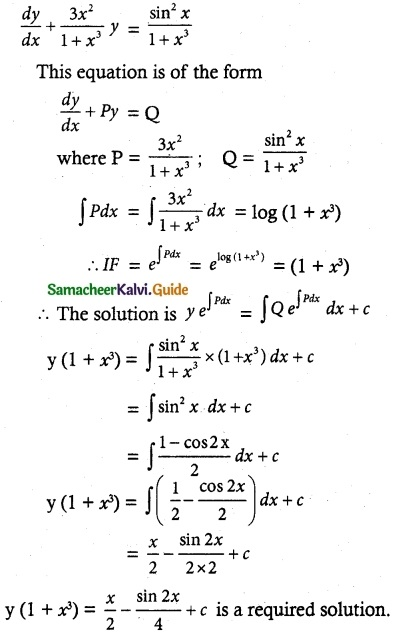Question 13.
x $$\frac { dy }{ dx }$$ + y = x log x
Solution:
The given differential equation may be written as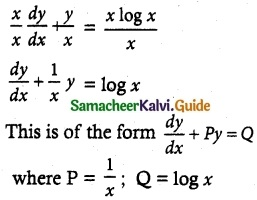Thus, the given differential equation is linear.
I.F = e∫pdx = e∫$$\frac { 1 }{ x }$$ dx = elog x = x
So, the solution of the given differential equation is given by
y × I.F = ∫(Q × I.F)dx + c
yx = ∫ log x x dx + c
yx = ∫ x log x dx + c
u = log x ∫dv = ∫x dxMultiply by 4
4yx = 2x² log x – x² + 4c
4xy = 2x² log x – x² + 4c is a required solution.Question 14.
x $$\frac { dy }{ dx }$$ + 2y – x² log x = 0
Solution:
The given differential equation may be written as
$$\frac { x }{ x }$$ $$\frac { dy }{ dx }$$ + $$\frac { 2y }{ x }$$ = $$\frac { x log x }{ x }$$
This is of the form $$\frac { dy }{ dx }$$ + Py = Q
where P = $$\frac { 2 }{ x }$$; Q = x log x
Thus, the given equation is linear.
I.F = e∫pdx = e∫$$\frac { 2 }{ x }$$ dx
elog x = elog x² = x²
So the required solution is
y × I.F = ∫(Q × I.F) dx + c
yx² = ∫x log x x² dx + c
yx² = ∫x³ logx dx + cMultiply by 16
16x²y = 4x4 log x – x4 + 16c is a required solutionQuestion 15.
$$\frac { dy }{ dx }$$ + $$\frac { 3y }{ x }$$ = $$\frac { 1 }{ x^2 }$$, given that y = 2 when x = 1
Solution:
The given differential equation can be written as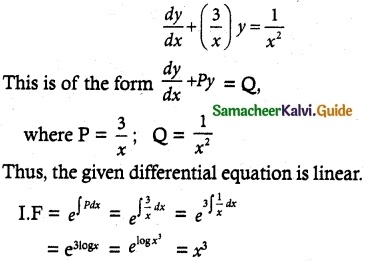So, its solution is given by
y × I.F = ∫(Q × I.F) dx + c
yx³ = ∫ $$\frac { 1 }{ x^2 }$$ + x³ dx + c
= ∫x dx + c
y x³ = $$\frac { x^2 }{ 2 }$$ + c
2yx³ = x² + c
Given that y = 2 when x = 1
2 (2) (1)³ = 1 + c
4 – 1 = c
c = 3
∴ 2yx³ = x² + 3 is a required solution.